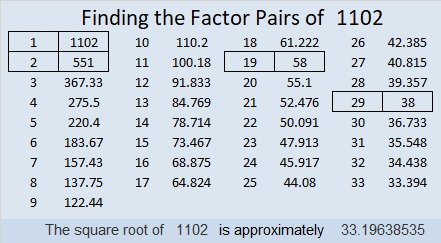# 1102 and Level 1

Write each number from 1 to 10 in both the first column and the top row so that those numbers are the factors of the given clues. This one is not too difficult, so if you haven’t solved one of these puzzles before, give it a try!Print the puzzles or type the solution in this excel file: 10-factors-1102-1110

Here is some information about the number 1102:

• 1102 is a composite number.
• Prime factorization: 1102 = 2 × 19 × 29
• The exponents in the prime factorization are 1, 1, and 1. Adding one to each and multiplying we get (1 + 1)(1 + 1)(1 + 1) = 2 × 2 × 2 = 8. Therefore 1102 has exactly 8 factors.
• Factors of 1102: 1, 2, 19, 29, 38, 58, 551, 1102
• Factor pairs: 1102 = 1 × 1102, 2 × 551, 19 × 58, or 29 × 38
• 1102 has no square factors that allow its square root to be simplified. √1102 ≈ 33.196391102 = 2(29)(19) so we know that 480-1102-1202 is a Pythagorean triple
calculated from 29²-19², 2(29)(19), 29²+19²

1102 is also the hypotenuse of a Pythagorean triple:
760-798-1102 which is (20-21-29) times 38.

1102 is a palindrome when it is written in a couple of other bases:
It’s 2F2 in BASE 20 (F is 15 base 10) because 2(20²) + 15(20) + 2(1) = 1102,
and it’s 262 in BASE 22 because 2(22²) + 6(22) + 2(1) = 1102.